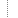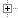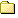Introduction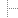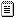What's NewPalettesUsing FormulasLayering in ChaosProRenderingFractal Parameter WindowsWindowsMenu3D TransformationsAnimationsFormula CompilerCompatibilityFractal Type Reference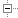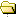Tutorials3D TransformationsAnimations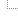Attractor Tutorial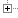Appendix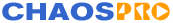Release 4.0Theory:

ChaosPro is able to automatically create animations (AVI files) or sequences of fractal pictures. The basic idea is as follows:

The user adds a fractal data structure (a data set which completely describes a fractal - FractInt users may think of a data structure as an entry in a *.par file) to the animation system. This data structure is copied and inserted into the list of keyframes.

1. The user then changes some parameters in the original data structure (for example, the number of iterations, parameter c, area values, rotation angle, etc.) and inserts the modified data set into the animation system again.
2. The user continues with this as required.
3. The user provides the animation system with some other parameters like, the size of the animation, the type (sequence of *.png files or an *.avi file), and specifies how many images should be calculated between the two key frames.
4. The user starts the process: ChaosPro subsequently creates fractal data structures, which interpolate the given keyframes, calculates the fractals described by the data structures, saves the image, and continues with the next part of the sequence.

For example let's assume the user subsequently adds keyframes with the following values:

• bailout=60, maxiter=80, horizontal view angle=30 degree
• bailout=80, maxiter=160, horizontal view angle=45 degree
• bailout=80, maxiter=200, horizontal view angle=70 degree
• bailout=70, maxiter=200, horizontal view angle=70 degree, zoomed in once (by double clicking on the centre of the window).

Assume, that the user chose linear interpolation and wants to calculate 4 frames from one keyframe to the next.

ChaosPro then examines these 4 keyframes (ChaosPro calls them animation keyframes or short: "AnimKeys"). Let us assume, that ChaosPro wants to set up frame number 11. The program sets up a function for the bailout value (as it changes during the sequence, ChaosPro will of course notice this without further interaction from the user). Below is an image describing how ChaosPro works.

The unit on the horizontal axis is the frame number, the vertical axis has the parameter values, i.e. the initial bailout values.

ChaosPro now fills in the values it knows, i.e. frame 1 has bailout=60, frame 5 (4 images should be calculated between 2 keyframes) has 80, frame 9 also has the value 80, and frame 13 has bailout=70.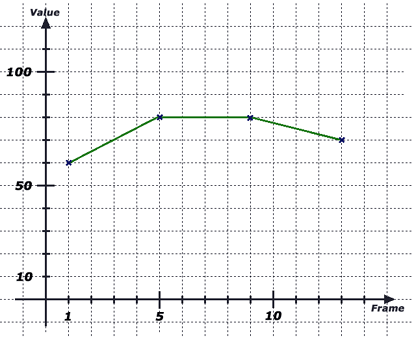The user chose linear interpolation, so a polygon is drawn to connect the 4 points. ChaosPro then extracts the value at point (image number) 11, which is 75, and this is how the bailout value for frame 11 is calculated.

Then the maximum number of iterations should be determined for frame number 11.

Again ChaosPro fills in the values known to the program, i.e. frame 1 has maxit=80, frame 5 has 160, frame 9 has the value 200, and frame 13 also has maxit=200.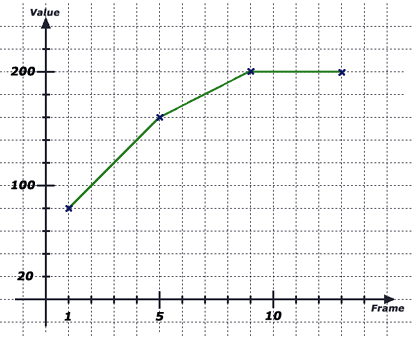As you can see, image number 11 has the value 200, so the iteration value for the data structure created when image 11 is calculated, will be 200.

The same method is applied to all parameters. ChaosPro examines the parameters, recognises whether the parameter changes and interpolates it. The interpolation type specifies how the points are connected. 'Linear' simply draws lines between them (as above), 'Spline' calculates a cubic spline (i.e. a smooth curve which interpolates the given points). Most of the time you will use 'Spline' as the interpolation type, as this generates smooth animations. The examples above use 'Linear' only for simplicity so that you can understand what is going on.

So you can for example calculate an animation, in which you look onto a 3D-transformed fractal (a kind of a landscape), then zoom into the fractal (you zoomed in on the 2D fractal), 'fly forward' (you moved the fractal around a little) while the palette changes and the fractal seems to morph (perhaps it was a juliaset and the user changed parameter C which gives some kind of morphing...) and at the end of the sequence the light source moves to the back and vanishes...

I hope that you recognise the power of this algorithm: Inside ChaosPro a fractal has a specific data structure of a predefined format where all relations to other "objects" like formulas are defined. A parameter can either belong to a fractal and thus resides in the fractal data structure, or it does not belong to a fractal and is therefore a global setting. This method of data separation makes it possible to compare the different fractal data structures and their parameters, and to create animations of almost all parameters in a fractal.

Note: You can't change all the parameters. Some of them act like switches (boolean values), and as you can imagine, there's no reasonable way to create sequences of images if a boolean value has changed. ChaosPro will detect it if you try to create such a sequence of images as soon as you try to add your fractal data structure to the animation.

Technique:

Now let me explain how I create animations, and how you should go about it:

1. Calculate a fractal which will define the starting point of the animation, and change it as you like.
2. As soon as you are satisfied with your changes, open the animation window (Menu: Windows / Animation), choose the "Key Frame Path" tab, and add the fractal to the keyframe list by clicking onto the button Add First. This is how a keyframe is created: All values, which describe the fractal, are copied into this keyframe structure. The values in this keyframe remain constant even if you change your fractal (because the values have been copied!).
3. Then change other parameters of the calculated fractal (the original one): Perhaps zoom in a bit, move it around, change the bailout value, or do something else (no, do NOT go away to drink some coffee!).
4. Then add it again (the same fractal, but now with other values...) to the keyframe list after the first one. (Select the first keyframe and click on the 'Add' button to insert the new keyframe directly after the current selection). ChaosPro is able to recognise the parameters which change between keyframes, and can create in-between data structures which interpolate from the parameter set of the first keyframe to the parameter set of the second keyframe. It should be obvious that one should not change too many parameters from one keyframe to the next, because the resulting fractal images will be far too dissimilar and thus the sequence will not resemble an animation. ChaosPro will still interpolate these using its algorithm, which works in every case, but if you do too many changes then you would have to calculate hundreds of frames between the two keyframes to get a smooth transition. I should zoom in about once (by a factor of two), then add this as a keyframe. If you want to change the parameters by a large amount, then do this bit by bit and add more keyframes to compensate.
5. Continue changing parameters and adding the fractal to the end of the keyframe list until you think you are finished. You can add as many keyframes as you like and you are only limited by the amount of memory available in your computer.
6. Once you have created the keyframes, you can define the width, height, and the number of frames to be calculated between your two (or more) keyframes.
7. When you have finished, click 'Start' in the keyframe tab of the animation window and wait for the animation creation to reach completion (this may take a while...).

Once upon a time somebody complained that creating animations didn't work like he expected. He did the following:

He took two fractal data sets and added them as keyframes. He presumed that ChaosPro would produce some kind of morphing from one fractal to the other but his two fractals were completely different. Although they were of a similar fractal type, one was a deep zoom into one location, whereas the other fractal was an even deeper zoom at another location. He specified 50 frames to be calculated between the two keyframes and wondered why this only produced black images. If he would have specified about 20,000,000 frames to calculate, this would have resulted in an animation lasting 188 hours (at 25 frames per second) and he would have seen that ChaosPro zoomed in a bit and then moved around before reaching its "goal", the second keyframe. The images were black, because ChaosPro had interpolated over the big "lake" of the Mandelbrot set, which was black in that case.

So my recommendation is: Don't try adding two fractal data structures as keyframes if you don't know exactly what you are doing. You won't crash ChaosPro (unless there is a bug), but you could easily get quite confused. Always make sure that the two keyframes don't have wildly different values, so the interpolation makes sense and produces reasonable values.

Of course, you can add two completely different keyframes. ChaosPro will interpolate in every case. And if you specify enough frames to calculate between the two keyframes, you will indeed get a smooth animation from the one keyframe to the other ...